Test: Sinusoidal Steady-State Analysis

# Test: Sinusoidal Steady-State Analysis

Test Description

## 10 Questions MCQ Test Topicwise Question Bank for Electrical Engineering | Test: Sinusoidal Steady-State Analysis

Test: Sinusoidal Steady-State Analysis for Electrical Engineering (EE) 2022 is part of Topicwise Question Bank for Electrical Engineering preparation. The Test: Sinusoidal Steady-State Analysis questions and answers have been prepared according to the Electrical Engineering (EE) exam syllabus.The Test: Sinusoidal Steady-State Analysis MCQs are made for Electrical Engineering (EE) 2022 Exam. Find important definitions, questions, notes, meanings, examples, exercises, MCQs and online tests for Test: Sinusoidal Steady-State Analysis below.
Solutions of Test: Sinusoidal Steady-State Analysis questions in English are available as part of our Topicwise Question Bank for Electrical Engineering for Electrical Engineering (EE) & Test: Sinusoidal Steady-State Analysis solutions in Hindi for Topicwise Question Bank for Electrical Engineering course. Download more important topics, notes, lectures and mock test series for Electrical Engineering (EE) Exam by signing up for free. Attempt Test: Sinusoidal Steady-State Analysis | 10 questions in 30 minutes | Mock test for Electrical Engineering (EE) preparation | Free important questions MCQ to study Topicwise Question Bank for Electrical Engineering for Electrical Engineering (EE) Exam | Download free PDF with solutions
 1 Crore+ students have signed up on EduRev. Have you?
Test: Sinusoidal Steady-State Analysis - Question 1

### The phasor diagram shown in figure below is for a two-element series circuit having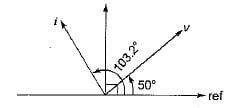Detailed Solution for Test: Sinusoidal Steady-State Analysis - Question 1

It is clear that i leads v by 53.2° (< 90°).
Hence the two series elements must be R and C.
∴ tan θ = tan 53.3°
tan 45° < tan 53.3° < tan 60° or,
1 < tan 53.3° <1.732
Hence, tan 53.3° = 1.3367

Test: Sinusoidal Steady-State Analysis - Question 2

### For the circuit shown below, the voltage across the 3 Ω resistor V3 is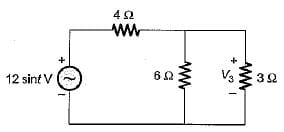Detailed Solution for Test: Sinusoidal Steady-State Analysis - Question 2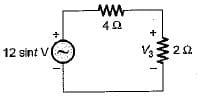Using voltage division rule, we have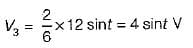Test: Sinusoidal Steady-State Analysis - Question 3

### A part of a circuit shown below consists of a resistor, a capacitor and an inductor. At steady state, iR(t) = 10 sin t and vL{t) = 5 cost. The rms value of the current through the capacitor is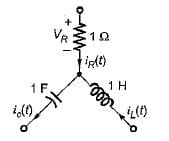Detailed Solution for Test: Sinusoidal Steady-State Analysis - Question 3

Using KCL at the given node,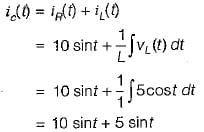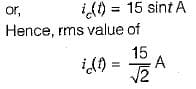Test: Sinusoidal Steady-State Analysis - Question 4

In the circuit shown below, currents I and I1 are respectively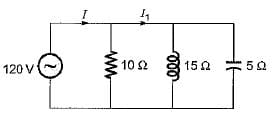Detailed Solution for Test: Sinusoidal Steady-State Analysis - Question 4

From given figure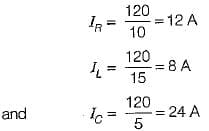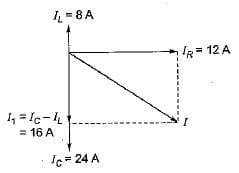Using phasor diagram shown below, the currents I and I1 are respectively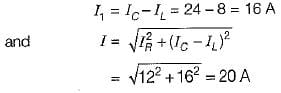Test: Sinusoidal Steady-State Analysis - Question 5

What should be the value of C for the circuit shown below such that the input power factor is unity for any frequency f of the source?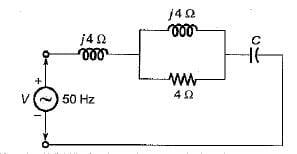Detailed Solution for Test: Sinusoidal Steady-State Analysis - Question 5

The input impedance Z of given circuit is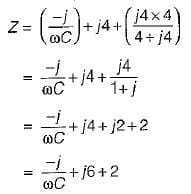For p.f. to be unity,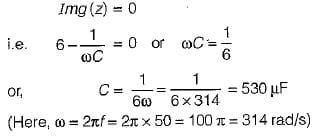Test: Sinusoidal Steady-State Analysis - Question 6

In a series R-L circuit, the current and voltages are given by
i = cos (314t-20°) , v = 10 cos ( 314 t + 10°). The values of R and L are respectively

Detailed Solution for Test: Sinusoidal Steady-State Analysis - Question 6

Here, i lags v by 30°

also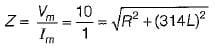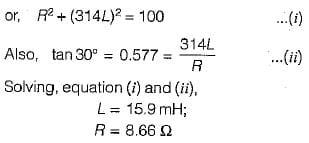Test: Sinusoidal Steady-State Analysis - Question 7

A series R-L circuit has resistance and reactance of 15 Ω and 10 Ω respectively. What should be the value of capacitor which when connected across the series combination in parallel, the system attains unity p.f.? (use f= 50 Hz)

Detailed Solution for Test: Sinusoidal Steady-State Analysis - Question 7

The situation is shown in figure below with it's phasor diagram.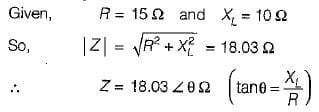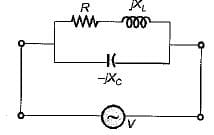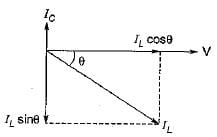For unity p.f. operation,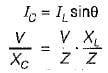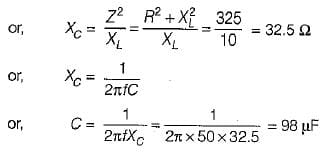Test: Sinusoidal Steady-State Analysis - Question 8

The input p.f. of the circuit shown below is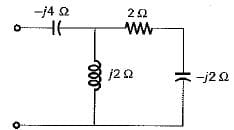Detailed Solution for Test: Sinusoidal Steady-State Analysis - Question 8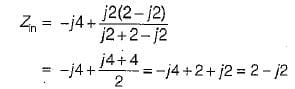or, Zin = 2.828∠-45°Ω
Hence, input p.f. = cos45° = 0.707 (lead)

Test: Sinusoidal Steady-State Analysis - Question 9

The input admittance of the circuit shown below is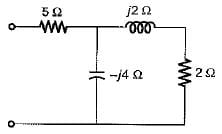Detailed Solution for Test: Sinusoidal Steady-State Analysis - Question 9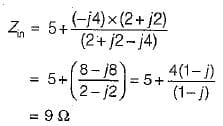Hence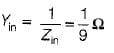Test: Sinusoidal Steady-State Analysis - Question 10

The driving point impedance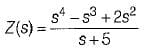does not represents a passive one port network because

## Topicwise Question Bank for Electrical Engineering

147 tests
 Use Code STAYHOME200 and get INR 200 additional OFF Use Coupon Code
Information about Test: Sinusoidal Steady-State Analysis Page
In this test you can find the Exam questions for Test: Sinusoidal Steady-State Analysis solved & explained in the simplest way possible. Besides giving Questions and answers for Test: Sinusoidal Steady-State Analysis, EduRev gives you an ample number of Online tests for practice

147 tests